# How to Use Go Generics?Are you aware of the fact that the lack of generics has been one of the most frustrating facts for Golang developers when writing a program? If you were to explore other blog posts, you would have found that developers had a tough time developing applications due to the absence of generics. But, much to the delight of everyone, Go 1.18 presented to us the implementation of generic features as per the specification of Type Parameter Proposal.
The inclusion of generics will definitely prompt more industry adoption in the coming years. But, before we delve into the programming aspect, let us understand what generics is all about.

## What is Generics in Go?

⦁Simply put, generics are useful for declaring and applying types and functions that are written down and coded, to work in conjunction with a particular set of types provided. We can write generic methods or functions where the type is determined when it is called or instantiated.
⦁What you need to understand is that in order to use the generics, you need to have the latest version of Go. (In my case, I’m using the version 1.19.2).

## What are the Use Cases of Generics?

⦁Now there is a highly polarizing issue in the Go community where some wanted it badly, while the others were not “missing” it. However, generics is a very powerful tool with very specific use cases. What you need to understand here is that not every library in Go will start implementing this generics.
⦁The most important use cases of generics involve math, data structures and algorithms. These in turn include stacks, queues, linked lists, etc.
⦁For example, you wish to add up a list of integers or floats or doubles. Now, without generics, you would have to create methods for each one of those. However, with generics, we can write this method only one time and use it for each of the types.

Meanwhile, if you are having issues with Golang application or microservice development, then you must not hesitate to reach out to Golang developers. All you have to do is get in touch with the experts at Golang.Company and the developers will cater to each and every requirement you have.

## How Does Generics Work in Golang?

⦁Next, we will take a look at how generics work in Golang. And this can be best understood in the form of programming. All the code will be accessible from the Github link https://github.com/GolangCompany/generics.

## Creating a New Directory

If you have been following the blog post series, you should be aware of the fact that for every new project, we create a directory. Let us name this directory ‘generics’. And I’ll use Command Prompt to create this as I’m on a Windows system. If you are using a Mac or Linux system, then you will have to use the Terminal.

`cd go-workspacemkdir genericscd genericscode .`With the last statement code ., the VS Code gets opened. You can use any other text editor or IDE of your choice.

### Getting Started with the Program

⦁Next, we will get started with the program. In order to do so, we will create a Go source file ‘main.go’ inside the directory ‘generics’. Now, in order to make this work, we will go to the Terminal of the VS Code and we will type: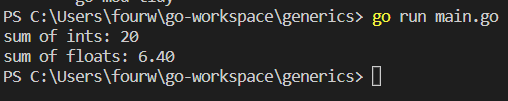This will lead to the creation of the go.mod file.
⦁And we will type the following program:
package main

`import ("constraints""fmt")type NumberType interface {int | float32 | float64 | uint16 | uint64 | uint32 | int32}func addGenerics[T constraints.Ordered](list []T) T {var sum Tfor _, item := range list {sum += item}return sum}func printAny(thing any) {fmt.Println(thing)}func Intsadd (list []int) int {var sum intfor _, item := range list {sum += item}return sum}func Floatsadd(list []float32) float32 {var sum float32for _, item := range list {sum += item}return sum}func main() {fmt.Printf("sum of ints: %d\n", Intsadd([]int{2, 3, 4, 5, 6}))fmt.Printf("sum of floats: %.2f\n", Floatsadd([]float32{1.2, 2.1, 3.1}))fmt.Printf("sum of ints: %d\n", addList([]int{2, 3, 4, 5, 6}))fmt.Printf("sum of floats: %.2f\n", addList([]float32{1.2, 2.1, 3.1}))printAny("hi")printAny(1.2)}`

### Dissecting the Program

⦁Now, we will try to comprehend the program. In this program we wanted to add a list of numbers of integers. So, we create a function Intsadd that is going to be a list of ints, and then it will return an int.
⦁Then we will create a sum variable for the integer and then we will loop through the list. For each item, we will add it to sum and finally, we will return sum
`func Intsadd (list []int) int {var sum intfor _, item := range list {sum += item}return sum}`

⦁Now, you might want to carry out the same operation of floats as well. So, what will we do? We will create a function and write down the same logic as the above function only with the function name Floatsadd which is going to be a list of float32, and it will return float32.
`func Floatsadd(list []float32) float32 {var sum float32for _, item := range list {sum += item}return sum}`

⦁Next, we go to the main function and we perform the following operation, i.e, print out the sum of integers and floats.
func main() {
fmt.Printf("sum of ints: %d\n", Intsadd([]int{2, 3, 4, 5, 6}))
fmt.Printf("sum of floats: %.2f\n", Floatsadd([]float32{1.2, 2.1, 3.1}))
}
So, let us check the output: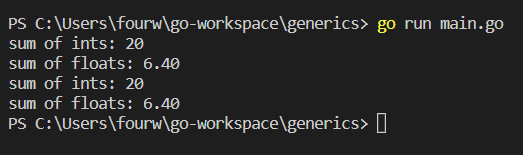As you can see, we have got the required output. But wasn’t that a lot of work? Writing different functions everytime. Here is where the generics come into the play.

## How to Implement the Generics?

⦁ So, let us create a Generics function first.

`func addGenerics[T int | float32](list []T) T {var sum Tfor _, item := range list {sum += item}return sum}`

So, we have the name of the method addGenerics, then open close brackets for the constraints. Let us call this type T int and use the OR operator to have more than one type and we will have float32. Then we will have the parameter list (list []T) and it will return T. Next, we will have the sum of type T and carry out the exact same operation as done in Intsadd and Floatsadd functions.
⦁Accordingly, we will write the main function as
`func main() {fmt.Printf("sum of ints: %d\n", Intsadd([]int{2, 3, 4, 5, 6}))fmt.Printf("sum of floats: %.2f\n", Floatsadd([]float32{1.2, 2.1, 3.1}))fmt.Printf("sum of ints: %d\n", addGenerics([]int{2, 3, 4, 5, 6}))fmt.Printf("sum of floats: %.2f\n", addGenerics([]float32{1.2, 2.1, 3.1}))}`
Now, let us check the output: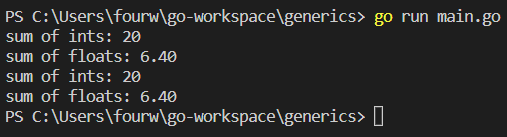Now, this too might be a bit cumbersome. This is especially true if you want to keep on adding different types like float64, uint16, etc. So, what we will do here is enforce the interface.

## How to Use Interface with Generics?

⦁In order to accomplish this, we will do the following:

type NumberType interface {
int | float32 | float64 | uint16 | uint64 | uint32 | int32
}
You can add as many types as you want.

⦁And you will make a slight adjustment in
`func addGenerics[T NumberType](list []T) T {var sum Tfor _, item := range list {sum += item}return sum}`
If you run the program, then this will work just fine: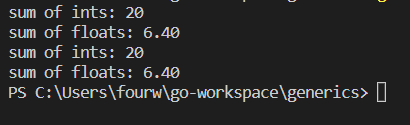## How to Use Constraints and Generics in Golang?

⦁There is a new keyword ‘any’ which is replacing an empty interface. But if you write it in place of an interface, there will be no constraint on any. So, any can represent anything. So, you can’t just add values of different types, because Go doesn’t know what it is. It requires a thorough check– a type check.
⦁Instead, what we can do is import a new package “constraints”. And after that, we will make changes in the addGenerics function.
func addGenerics[T constraints.Ordered](list []T) T {
var sum T
for _, item := range list {
sum += item
}
return sum
}

The constraints.Ordered will allow any number type that is going to be added or compared. Now that we have the program at hand, let us check for the output.## Taking a Closer Look at any Keyword

⦁In this section, we will take a look at the ‘any’ keyword. For this, Let us jump right into the program. We write a function func Any (thing any).

func printAny(thing any) {
fmt.Println(thing)
}
Accordingly we include the statement in the main function
func main()
{
printAny("hi")
printAny(1.2)

}
Now, let us have a look at the output: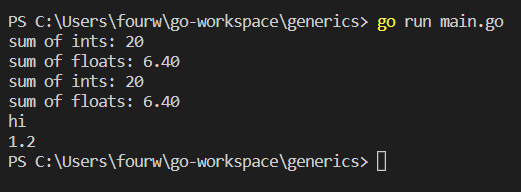As you can see, we have the desired result.
Hopefully, you have understood the concept of generics in Golang. Now, you might not have immediate usage in the language if you are accustomed to the interface. But, sooner or later, you might require it. Most importantly, it leads to the reduction in boilerplate. And who doesn’t want that?Soham Mukherjee

I have been working as a content writer for four years, after I graduated in 2018 with a B.Tech in Electronics and Telecommunication. Learning about programming languages and technologies is a passion for me. Apart from programming, I like to play guitar in my leisure time.